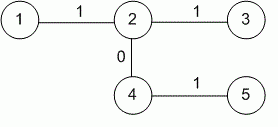Problems

# XOR Sum

A tree with n vertices is given. The edges of the tree have a weight of 0 or 1 only. Find the XOR sum between all pairs of vertices. Compute the sum of all XOR sums.

#### Input

The first line contains the number of vertices n (2n105) in graph. It is followed by n - 1 lines containing the description of edges. Every line of description consists of three integer numbers: vertices connected by the edge (labelled from 1 to n) and edge's weight (0 or 1).

#### Output

Print the sum of XOR sums between the pairs of vertices.Time limit 1 second
Memory limit 128 MiB
Input example #1
5
1 2 1
2 3 1
2 4 0
4 5 1

Output example #1
6

Author Michael Medvediev